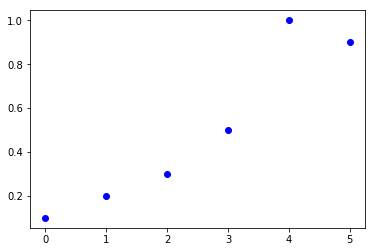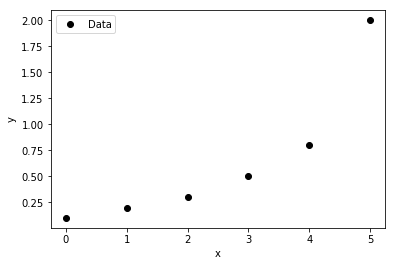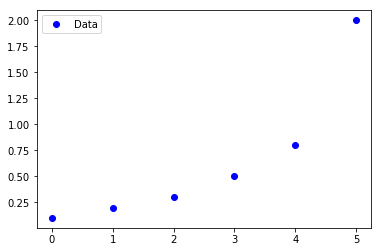## GEKKO Python Example Applications¶GEKKO is optimization software for mixed-integer and differential algebraic equations. It is coupled with large-scale solvers for linear, quadratic, nonlinear, and mixed integer programming (LP, QP, NLP, MILP, MINLP). Modes of operation include data reconciliation, real-time optimization, dynamic simulation, and nonlinear predictive control. See the GEKKO documentation for additional information.

1. Solver Selection
2. Solve Linear Equations
3. Solve Nonlinear Equations
4. Interpolation with Cubic Spline
5. Linear and Polynomial Regression
6. Nonlinear Regression
7. Machine Learning / Artificial Neural Network
8. Solve Differential Equation(s)
9. Nonlinear Programming Optimization
10. Mixed Integer Nonlinear Programming
11. Optimal Control with Integral Objective
12. Optimal Control with Economic Objective
13. Optimal Control: Minimize Final Time
14. PID Control Tuning
15. Process Simulator
16. Moving Horizon Estimation
17. Model Predictive Control
18. Debugging Resources

This is a blank workbook that is filled in with solutions available on GitHub or through an online notebook viewer.

In :
# install and import GEKKO

# other packages needed in this notebook
import numpy as np
import matplotlib.pyplot as plt
%matplotlib inline


## 1: Solver selection¶

Solve $y^2=1$ with APOPT solver. See APMonitor documentation or GEKKO documentation for additional solver options.

In [ ]:



## 2: Solve Linear Equations¶

$3\,x + 2\,y=1$

$x+2\,y=0$

In [ ]:



## 3: Solve Nonlinear Equations¶

$x+2\,y=0$

$x^2+y^2=1$

In [ ]:



## 4: Interpolation with Cubic Spline¶

In :
xm = np.array([0,1,2,3,4,5])
ym = np.array([0.1,0.2,0.3,0.5,1.0,0.9])

# create plot
plt.plot(xm,ym,'bo')
plt.legend(loc='best')## 5: Linear and Polynomial Regression¶

In :
xm = np.array([0,1,2,3,4,5])
ym = np.array([0.1,0.2,0.3,0.5,0.8,2.0])

# plot data
plt.plot(xm,ym,'ko',label='Data')
plt.legend(loc='best')
plt.xlabel('x')
plt.ylabel('y')

Out:
Text(0,0.5,'y')## 6: Nonlinear Regression¶

In :
# measurements
xm = np.array([0,1,2,3,4,5])
ym = np.array([0.1,0.2,0.3,0.5,0.8,2.0])

plt.plot(xm,ym,'bo',label='Data')
plt.legend()

Out:
<matplotlib.legend.Legend at 0x1acfa0278d0>## 7: Machine Learning¶

Approximate $y = sin(x)$ with an Artificial Neural Network

Trigonometric Function (trig=True)

• Input: $x$
• Layer 1: linear layer, 1 node, $l1 = w1 \; x$
• Layer 2: nonlinear layer, 1 node, cosine function, $l2 = \cos(w2a+w2b\;l1)$
• Layer 3: linear layer, 1 node, $l3 = w3 \; l2$
• Output: $y = \sum{l3}$

Artificial Neural Network Description (trig=False)

• Input: $x$
• Layer 1: linear layer, 2 nodes, $l1 = w1 \; x$
• Layer 2: nonlinear layer, 2 nodes, hyperbolic tangent activation function, $l2 = \tanh(w2a+w2b\;l1)$
• Layer 3: linear layer, 2 nodes, $l3 = w3 \; l2$
• Output: $y = \sum{l3}$

See Online Neural Network Demo with TensorFlow.

In [ ]:



## 8: Solve Differential Equation(s)¶

Solve the following differential equation with initial condition $y(0) = 5$:

$k \, \frac{dy}{dt} = -t \, y$

where $k=10$. The solution of $y(t)$ should be reported from an initial time $0$ to final time $20$. Create of plot of the result for $y(t)$ versus $t$.

In [ ]:



## 9: Nonlinear Programming Optimization¶

Solve the following nonlinear optimization problem:

$\min x_1 x_4 \left(x_1 + x_2 + x_3\right) + x_3$

$\mathrm{s.t.} \quad x_1 x_2 x_3 x_4 \ge 25$

$x_1^2 + x_2^2 + x_3^2 + x_4^2 = 40$

$1\le x_1, x_2, x_3, x_4 \le 5$

with initial conditions:

$x_0 = (1,5,5,1)$

In [ ]:



## 10: Mixed Integer Nonlinear Programming¶

In [ ]:



## 11: Optimal Control with Integral Objective¶

Original Form

$\min_u \frac{1}{2} \int_0^2 x_1^2(t) \, dt$

$\mathrm{subject \; to}$

$\frac{dx_1}{dt}=u$

$x_1(0) = 1$

$-1 \le u(t) \le 1$

Equivalent Form for GEKKO with new Variable $x_2$

$\min_u x_2\left(t_f\right)$

$\mathrm{subject \; to}$

$\frac{dx_1}{dt}=u$

$\frac{dx_2}{dt} = \frac{1}{2} x_1^2(t)$

$x_1(0) = 1$

$x_2(0) = 0$

$t_f = 2$

$-1 \le u(t) \le 1$

In [ ]:



## 12: Optimal Control with Economic Objective¶

Original Form

$\max_{u(t)} \int_0^{10} \left(E-\frac{c}{x}\right) u \, U_{max} \, dt$

$\mathrm{subject \; to}$

$\frac{dx}{dt}=r \, x(t) \left(1-\frac{x(t)}{k}\right)-u \, U_{max}$

$x(0) = 70$

$0 \le u(t) \le 1$

$E=1, \, c=17.5, \, r=0.71$

$k=80.5, \, U_{max}=20$

Equivalent Form for GEKKO

$\min_{u(t)} -J\left(t_f\right)$

$\mathrm{subject \; to}$

$\frac{dx}{dt}=r \, x(t) \left(1-\frac{x(t)}{k}\right)-u \, U_{max}$

$\frac{dJ}{dt} = \left(E-\frac{c}{x}\right) u \, U_{max}$

$x(0) = 70$

$J(0) = 0$

$0 \le u(t) \le 1$

$t_f = 10, \, E=1, \, c=17.5$

$r=0.71, \, k=80.5, \, U_{max}=20$

In [ ]:



## 13: Optimal Control: Minimize Final Time¶

Original Form

$\min_{u(t)} \; t_f$

$\mathrm{subject \; to}$

$\frac{dx_1}{dt}=u$

$\frac{dx_2}{dt}=\cos\left(x_1(t)\right)$

$\frac{dx_3}{dt}=\sin\left(x_1(t)\right)$

$x(0) = \left[\pi/2,4,0\right]$

$x_2\left(t_f\right)=0$

$x_3\left(t_f\right)=0$

$-2 \le u(t) \le 2$

Equivalent Form for GEKKO

$\min_{u(t), t_f} \; t_f$

$\mathrm{subject \; to}$

$\frac{dx_1}{dt}=t_f \, u$

$\frac{dx_2}{dt}=t_f \, \cos\left(x_1(t)\right)$

$\frac{dx_3}{dt}=t_f \, \sin\left(x_1(t)\right)$

$x(0) = \left[\pi/2,4,0\right]$

$x_2\left(t_f\right)=0$

$x_3\left(t_f\right)=0$

$-2 \le u(t) \le 2$

In [ ]:



## 14: PID Control Tuning¶

A PID Controller has proportional, integral, and derivative terms to determine the controller output ($OP$) based on the set point ($SP$) and process variable ($PV$). A standard PID form has constants $K_c$, $\tau_I$, and $\tau_D$.

$err = SP-PV$

$OP = OP_0 + K_c \, err + \frac{K_c}{\tau_I} \int err \, dt - K_c \, \tau_D \frac{d\,PV}{dt}$

The effect of the tuning constants is shown with the PID Tuning Notebook. This example is an alternative implementation in GEKKO.

In [ ]:



## 15: Process Simulator¶

In [ ]:



## 16: Moving Horizon Estimation¶

Run the Process Simulation cell above to generate the data. The MHE application uses a first order model while the process simulation is a second order system. This is done to emulate a realistic case with model mismatch and measurement noise.

This demonstrates just one cycle of an MHE application. Typical MHE applications receive an additional measurements, re-optimize parameters and states, and re-inject the parameters into a controller.

In [ ]:



## 17: Model Predictive Control¶

In [ ]:



## 18: Debugging Resources¶

Applications may need a more detailed inspection to find errors in programming syntax, errors in modeling assumptions, or to generate good initial guess values. The GEKKO or the solver solution reports syntax errors. Setting m.solve(disp=True) displays the solver output with a message on the line of code that is unsuccessful. Naming the variables such as name='state' is helpful to display the equations in a readable form.

Other strategies for obtaining a successful solution include:

• Increase the number of iterations with MAX_ITER (0-1000+)
• Increase the diagnostic level with DIAGLEVEL (0-10)
• Change the solver with SOLVER (1-5)
• Calculate model SENSITIVITY (1)
• Solve a square problem with # Variables = # Equations
• Set COLDSTART to initialize problem (0-2)

Additional modeling, initialization, and decomposition tips may be helpful. There is also an online discussion group, video playlist, GEKKO documentation, and APMonitor documentation as additional resources.

In [ ]: